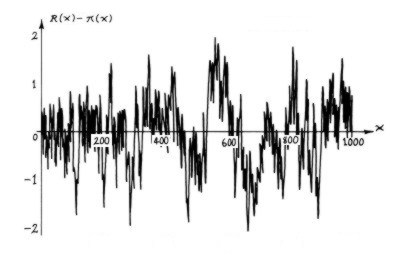It seems, then, that the distribution of prime numbers "points to" or implies Riemann's function R(x). This function can be thought of as a smooth ideal to which the actual, jagged, prime counting function clings. The next layer of information contained in the primes can be seen above, which is the result of subtracting π(x) from R(x). This function relates directly to the local fluctuations of the density of primes from their mean density. In their article "Are prime numbers regularly ordered?", three Argentinian chaos theorists considered this function, treated it as a "signal", and calculated its Liapunov exponents. These are generally computed for signals originating with physical phenomena, and allow one to decide whether or not the underlying mechanism is chaotic. The authors conclude "...a regular pattern describing the prime number distribution cannot be found. Also, from a physical point of view, we can say that any physical system whose dynamics is unknown but isomorphic to the prime number distribution has a chaotic behaviour." A physicist shown the above graph might naturally think to attempt a Fourier analysis – i.e. to see if this noisy signal can be decomposed into a number of periodic sine-wave functions. In fact something very much like this is possible. To understand how, we must look at the Riemann zeta function. << previous      beginning      next >>